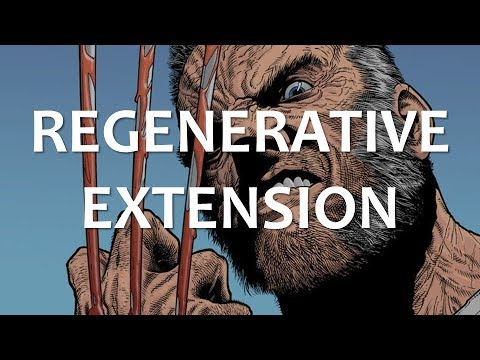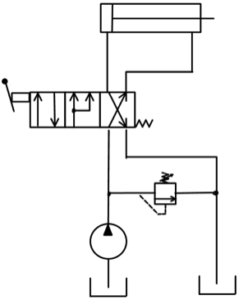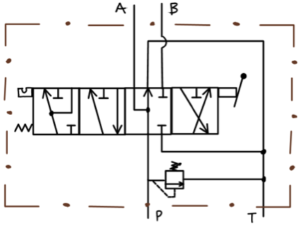# 3.5: Regenerative ExtensionA YouTube element has been excluded from this version of the text. You can view it online here: https://openoregon.pressbooks.pub/hydraulics/?p=86

Given volume (V) and time (t) solve for flow rate (Q).

Given time (t) and flow rate (Q) solve for volume (V).

Given flow rate (Q) and time (t) solve for volume (V).

Given a cylinder with the following dimensions:

dcap =2 ½ in

drod = 1 in

travel = 24 in

Calculate the time to fully extend the cylinder and the time to fully retract the cylinder in units of seconds given a constant flow rate of 2.3 gpm. Additionally, calculate maximum extension and retraction force given maximum pressure of 400psi.

Determine the response of a double acting cylinder given equal pressure on the cap and rod end. Use Pascal’s Law to explain your answer.

Determine the force and functional area imbalance between cap and rod end of the above cylinder given equal pressure on the cap and rod end.

Describe flow patterns within a double acting cylinder in regenerative extension mode.

Identify the volume differential between the cap end at full extension and the rod end at full retraction.

Compare the extension force and speed for a cylinder in regenerative extension mode with one in normal extension mode.

Given the above example cylinder calculate the extension speed in units of seconds while in regenerative extension mode.

Given a cylinder with the following dimensions and a system with the following parameters:

dcap =3 in

drod = 1 ¼ in

travel = 16 in

Q = 1.8gpm

Pmax = 500psi

Calculate the time to fully extend the cylinder the cylinder in units of seconds and maximum extension force in normal extension mode:

Calculate the time to fully extend the cylinder the cylinder in units of seconds and maximum extension force in regenerative extension mode:

Describe applications for regenerative extension.

Describe the physical characteristics of a 2:1 cylinder and its application in a system that routes pressurized flow to both cap and rod end serving to extend the cylinder and a cross connect position serving to retract the cylinder.

Given a 2:1 cylinder with the following dimensions:

dcap =1 ½ in

drod = 1.06066017178 in

travel = 12 in

Q = 0.9gpm

Pmax = 700psi

Calculate the extension and retraction force and the time to fully extend and retract given extension in regenerative mode.

Describe the operation of this system employing a manually actuated 3 position directional control valve spring offset into the cross connect position with a soft and hard stop.Describe the purpose of each position for this directional control valve.Describe the operation of this system employing additional directional control valves.